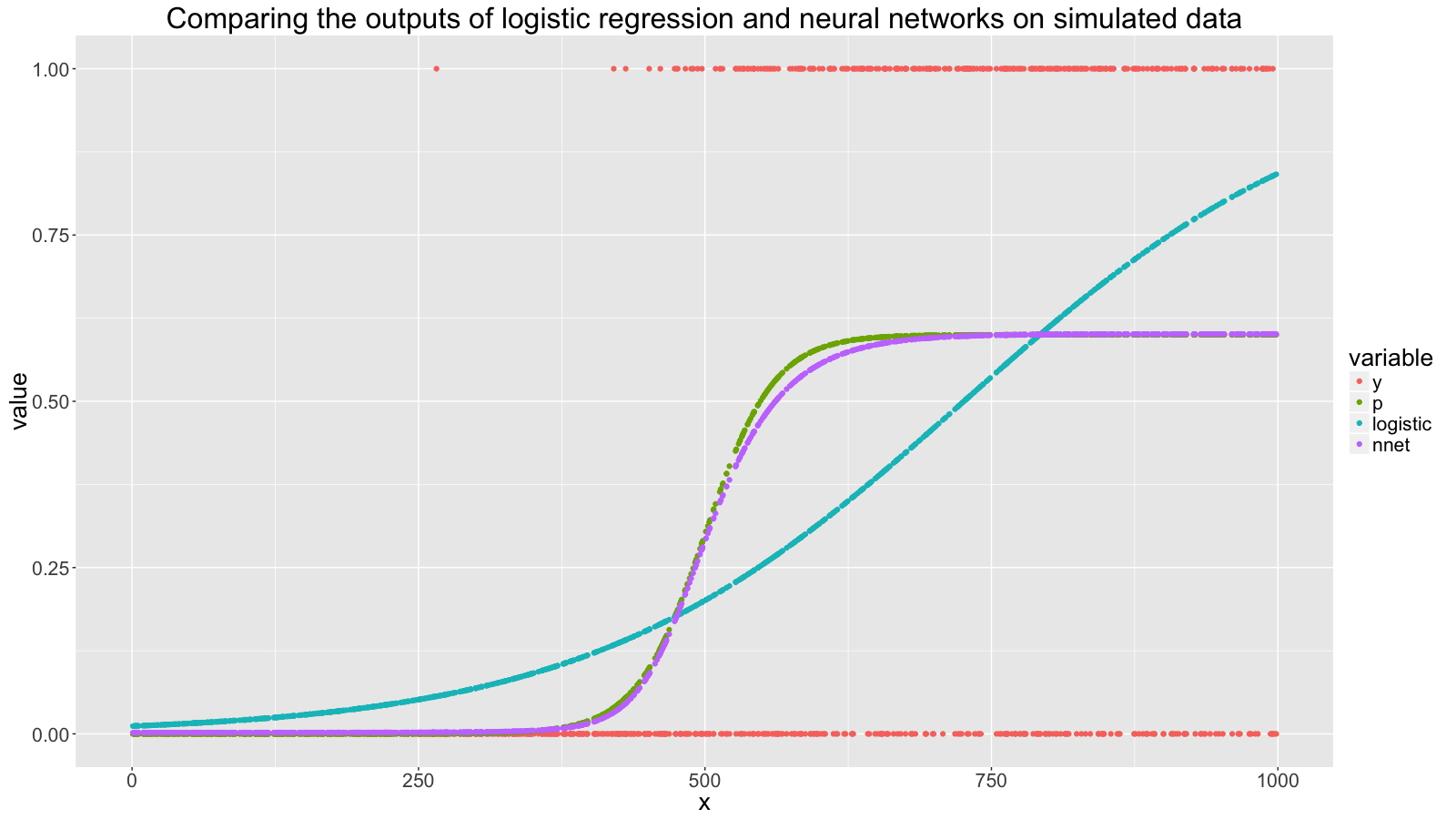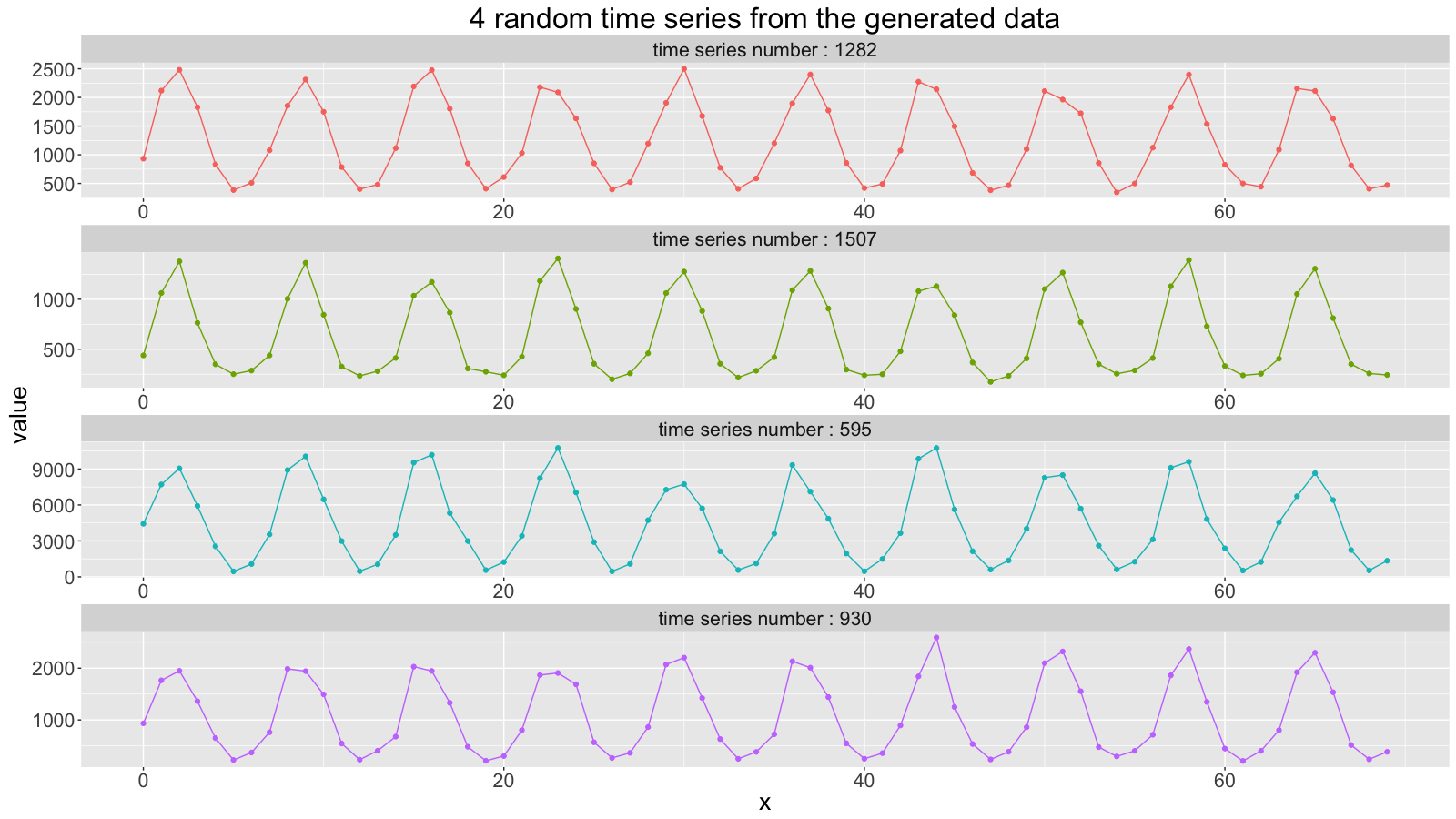# Generating Data in R

## The Introduction

In a previous post on A/B Tests I used rbinom() to generate some example data. This post looks at similar functions, extends their usefulness with Vectorize() and details how we commonly use these functions at uSwitch.

## The Uses

Here are some things that uSwitch analysts use R’s data generation functions for:

#### Generating demonstrative examples or teaching examples:

At uSwitch, we find time to teach each other. Often, when teaching the basics about a particular function or statistical method, it is easiest to do so with simulated data.

This has three benefits over real data:

• it’s as simple as we want it to be
• it doesn’t require cleaning
• the student can view exactly how the data is generated

#### Testing data transformations:

When building data transformations or statistical methods, we sometimes want to see how the transformation responds to specific situations. As in the A/B tests blogpost, we used data generation to check that the prioritiser would prioritise the mobile segment as the most important to look at.

#### Analysing risk in A/B tests:

With the results of a split test with a success/fail outcome variable and a prior (usually uniform) distribution, we can sample from the posterior distribution of success rates and answer questions like ‘Assuming an uninformative prior, what proportion of the time would we expect variant A to perform 0.1 percentage point better than variant B?’

## The Functions

These functions generate vectors of samples from a specified distribution:
sample(), rbinom(), rbeta(), runif(), rnorm(), rpois()
There are more in base R, but these are the ones we’ve found most useful.

sample(): Used for generating samples from a specified vector
rbinom(), runif(), rbeta(), rnorm(), rpois(): used for sampling from binomial, uniform, beta, normal and poisson distributions, respectively.

Binomial distributions can be used for generating binary outcome data from observations with a fixed chance of success.
Beta distributions can be used for generating probabilities between 0 and 1, and often useful for a prior and posterior distribution in Bayesian analysis of A/B tests. Poisson distributions can be used to generate count data based on a constant average count per unit time.

## The Extensions

We can easily extend the rbinom(), rbeta(), runif(), rnorm() and rpois() functions so that they can take a vector for each parameter and output a vector of samples: one for each distribution specified by each row.
We write some new functions which will generate samples from a distribution with varying parameters, taking as input a vector for each parameter rather than a single number.

This is a bit abstract, so here is an example:

``````#This will generate 5 samples from a uniform distribution between 0 and 10.
runif(5, min = 0, max = 10)

#We can extend the runif() function like this:
runif_vec_proto <- function(min, max) {return(runif(1, min = min, max = max))}
runif_vec <- Vectorize(runif_vec_proto); runif_vec_proto <- NULL

#Using this new runif_vec() function we can run this:
runif_vec(min = c(0, 0, 3, 3, 7), max = c(5 , 5, 6, 6, 10))
#Which will generate a vector with two samples from the uniform distribution
#between 0 and 5, two samples from the uniform distribution between 3 and 6
#and one sample from the uniform distribution between 7 and 10.
``````

These extensions help us when we want to simulate a diverse and varied dataset.

We’ll write some more extensions in the second and third examples in the next section.

## The Examples

#### First Example

Using some example A/B test results and rbeta(), we’ll estimate the chance that the true success rate of B is higher than that of A.

``````#example results
observations_in_A <- 5000; observations_in_B <- 5000;
successes_in_A <- 510; successes_in_B <- 553

#success rates
successes_in_A/observations_in_A;successes_in_B/observations_in_B;

#fails calculations for beta distribution
fails_in_A <- observations_in_A - successes_in_A
fails_in_B <- observations_in_B - successes_in_B

#Sample from the posterior distribution for each variant, assuming uninformative prior:
samples <- 1000000
sampled_true_success_rates_A <- rbeta(samples, successes_in_A+1, fails_in_A+1)
sampled_true_success_rates_B <- rbeta(samples, successes_in_B+1, fails_in_B+1)
sampled_absolute_increase_in_BvsA <- sampled_true_success_rates_B-sampled_true_success_rates_A

#Look at the quantiles, for interest
quantile(sampled_absolute_increase_in_BvsA, seq(0,1, 0.05))

#Estimate the chance that the true success rate of B is greater than that of A
mean(sampled_absolute_increase_in_BvsA>0)
``````

#### Second Example

We’ll generate some success/fail outcome data with probability depending on an S curve over a real independent variable, in order to compare the results of logistic regression versus a simple single-layer, single-hidden-layer neural network.

``````#install.packages(c(‘dplyr’, ‘nnet’, ‘ggplot2’, 'reshape2'))
require(dplyr); require(nnet); require(ggplot2); require(reshape2)

#generate independent variable samples
x <- runif(1000, 0, 1000)

#generate success/fail observations, which has a probability which follows
#a logistic curve which is close to 0 around x = 0,
#close to 0.6 around x=1000, and close to 0.3 around x=500
d <- 0.6*plogis(x, location = 500, scale = 30)
rbinom_vec_proto <- function(p, size = 1) {return(rbinom(1, size, p))}
rbinom_vec <- Vectorize(rbinom_vec_proto); rbinom_vec_proto <- NULL
y <- rbinom_vec(d)

data <- data.frame(x = x,  y = y)

#Add the fitted values from standard logistic regression and from a single neuron neural network to the data frame
m <- glm(y~x, data, family = binomial())
f <- m\$fitted.values
m2 <- nnet(y~x, data, size = 1, entropy = TRUE, abstol = 1.0e-7, reltol = 1.0e-10)
f2 <- m2\$fitted.values
data  <- data.frame(x = x, y = y, p = d, logistic = f, nnet = f2)

#Plot the original outcome probability, the binary outcome data,
#and the fitted values from our two models for each x:
data %>% melt('x') %>% ggplot(aes(x, value, color = variable)) + geom_point()
``````#### Third Example

As a prelude to a future blog post on anomaly detection in time series, we’ll simulate hundreds of time series of traffic using Poisson and Uniform distributions:

``````#install.packages(c(‘dplyr’, ‘ggplot2’))
require(dplyr); require(ggplot2)

#write our function extensions
rpois_vec_proto <- function(lambda) {return(rpois(1, lambda = lambda))}
rpois_vec <- Vectorize(rpois_vec_proto)
runif_vec_proto <- function(min, max) {return(runif(1, min = min, max = max))}
runif_vec <- Vectorize(runif_vec_proto)

#Parameters
week_length <- 7
days <- 10*week_length
day_numbers <- 0:(days-1)
weekly_average_range <- c(10, 10000)
ts_count = 1600

#generate weekly averages
weekly_averages <- rep(runif(n = ts_count, weekly_average_range, weekly_average_range), each = days)

#to each time series. We’ll use these in a moment
min_below_fraction <- rep(runif(n = ts_count, 0.25, 1), each = days)
max_above_fraction <- rep(runif(n = ts_count, 1, 2), each = days)
unif_maxadd_fraction <- rep(runif(n = ts_count, 0, 0.7), each = days)

#Add these into a data frame
time_series <- data.frame(
ts_number = rep(1:ts_count, each = days),
day_number = rep(day_numbers, times = ts_count),
min_below_fraction = min_below_fraction, max_above_fraction = max_above_fraction,
weekly_averages = weekly_averages
)

#Build up the time series
time_series <-
#Use the sin function to generate cyclic sinusoidal shape, according to the length of the week
mutate(time_series, cycle_unstretched_shape = sin(2*pi*day_number/week_length)) %>%
#Stretch this using our randomly generated attributes
mutate(cycle_stretched_shape = ifelse(cycle_unstretched_shape <= 0,
cycle_unstretched_shape*min_below_fraction,
cycle_unstretched_shape*max_above_fraction)) %>%
#Get the average per day, according to what day of the week it is
mutate(cyclic_averages = weekly_averages*(1+cycle_stretched_shape)) %>%
#Get the actual count via Poisson distribution
mutate(poisson_count = rpois_vec(cyclic_averages)) %>%
#Add a random uniformly generated count

time_series_2 <- select(time_series, ts_number, day_number, count = unif_add_count)

#Chart 4 random series
time_series_2[time_series_2\$ts_number %in% sample(ts_count, 4),] %>%
ggplot(aes(day_number, count)) + geom_point() + geom_line() +
facet_wrap(~ts_number, ncol = 1, scale = 'free')
``````## The Conclusion

The code we’ve used here delves into an area that goes by many names: Bayesian Networks, Probabilistic Graphical Models and Bayesian Hierarchical Models are a few. For more complicated generative models, I would recommend a different approach to the one outlined above. One approach I am familiar with uses the pymc package in Python, an excellent introduction of which can be found in Cam Davidson-Pilon’s ebook Probabilistic Programming and Bayesian Methods for Hackers.

For simple data generation however we find the functions in this post quick and easy to use. We hope you find them useful too.